# Chapter 11 Approximation Algorithms Slides by Kevin Wayne

• Slides: 60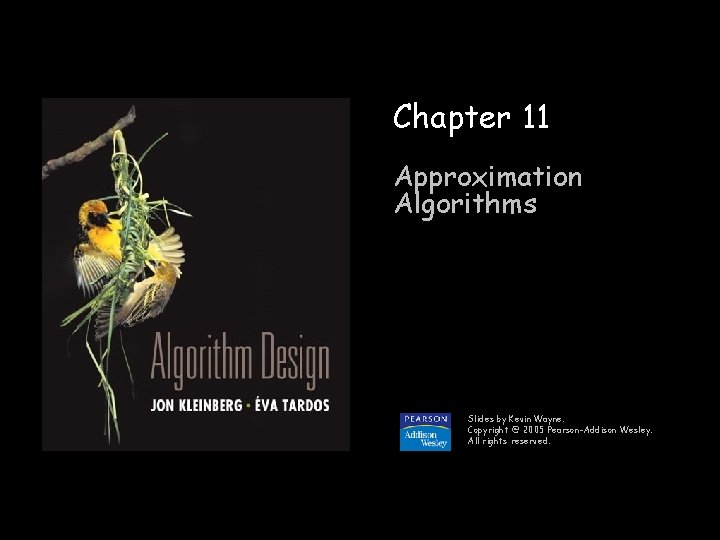Approximation Algorithms Q. Suppose I need to solve an NP-hard problem. What should I do? A. Theory says you're unlikely to find a poly-time algorithm. Must sacrifice one of three desired features. Solve problem to optimality. Solve problem in poly-time. Solve arbitrary instances of the problem. n n n -approximation algorithm. Guaranteed to run in poly-time. Guaranteed to solve arbitrary instance of the problem Guaranteed to find solution within ratio of true optimum. n n n Challenge. Need to prove a solution's value is close to optimum, without even knowing what optimum value is! 2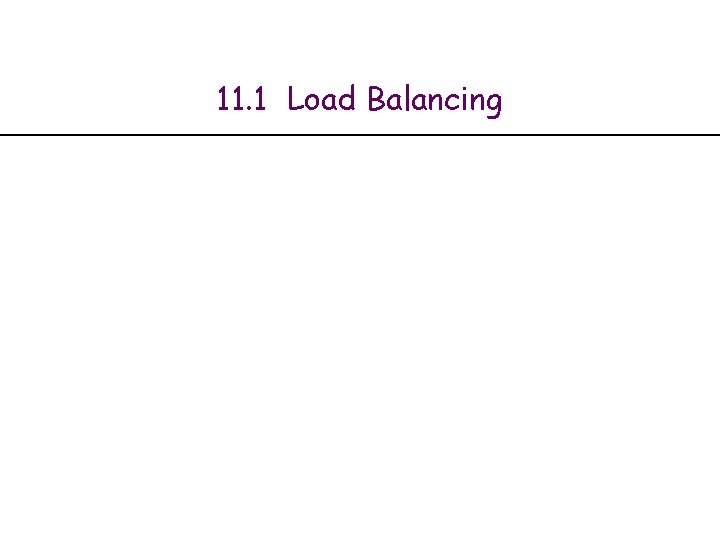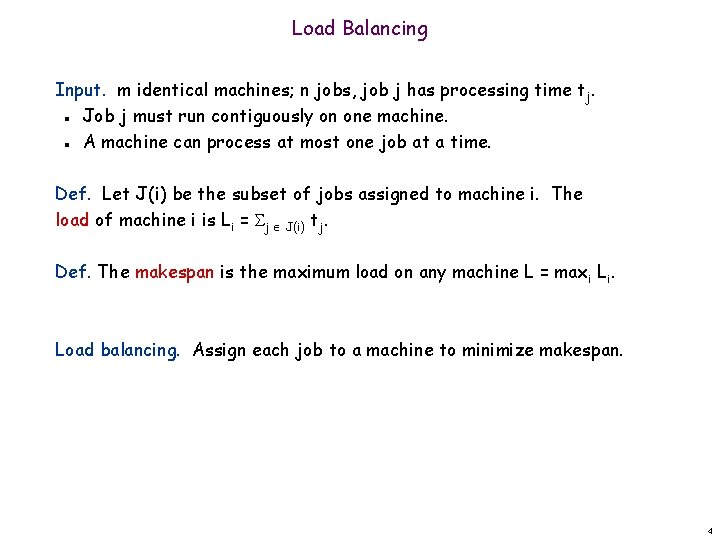Load Balancing Input. m identical machines; n jobs, job j has processing time tj. Job j must run contiguously on one machine. A machine can process at most one job at a time. n n Def. Let J(i) be the subset of jobs assigned to machine i. The load of machine i is Li = j J(i) tj. Def. The makespan is the maximum load on any machine L = maxi Li. Load balancing. Assign each job to a machine to minimize makespan. 4Load Balancing: List Scheduling List-scheduling algorithm. Consider n jobs in some fixed order. Assign job j to machine whose load is smallest so far. n n List-Scheduling(m, n, t 1, t 2, …, tn) { for i = 1 to m { load on machine i Li 0 jobs assigned to machine i J(i) } for j = 1 to n { i = argmink Lk J(i) {j} L i + tj } machine i has smallest load assign job j to machine i update load of machine i } Implementation. O(n log n) using a priority queue. 5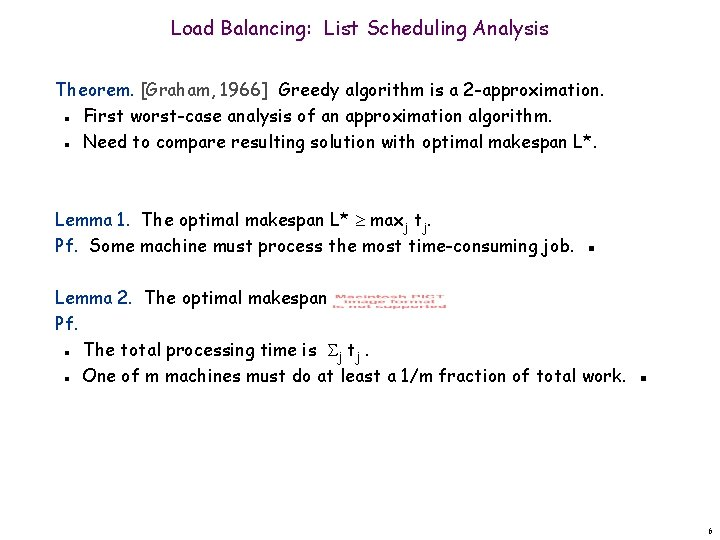Load Balancing: List Scheduling Analysis Theorem. [Graham, 1966] Greedy algorithm is a 2 -approximation. First worst-case analysis of an approximation algorithm. Need to compare resulting solution with optimal makespan L*. n n Lemma 1. The optimal makespan L* maxj tj. Pf. Some machine must process the most time-consuming job. ▪ Lemma 2. The optimal makespan Pf. The total processing time is j tj. One of m machines must do at least a 1/m fraction of total work. ▪ n n 6Load Balancing: List Scheduling Analysis Theorem. Greedy algorithm is a 2 -approximation. Pf. Consider load Li of bottleneck machine i. Let j be last job scheduled on machine i. When job j assigned to machine i, i had smallest load. Its load before assignment is Li - tj Lk for all 1 k m. n n blue jobs scheduled before j machine i j 0 L i - tj L = Li 7Load Balancing: List Scheduling Analysis Theorem. Greedy algorithm is a 2 -approximation. Pf. Consider load Li of bottleneck machine i. Let j be last job scheduled on machine i. When job j assigned to machine i, i had smallest load. Its load before assignment is Li - tj Lk for all 1 k m. Sum inequalities over all k and divide by m: n n n Lemma 1 n Now ▪ Lemma 2 8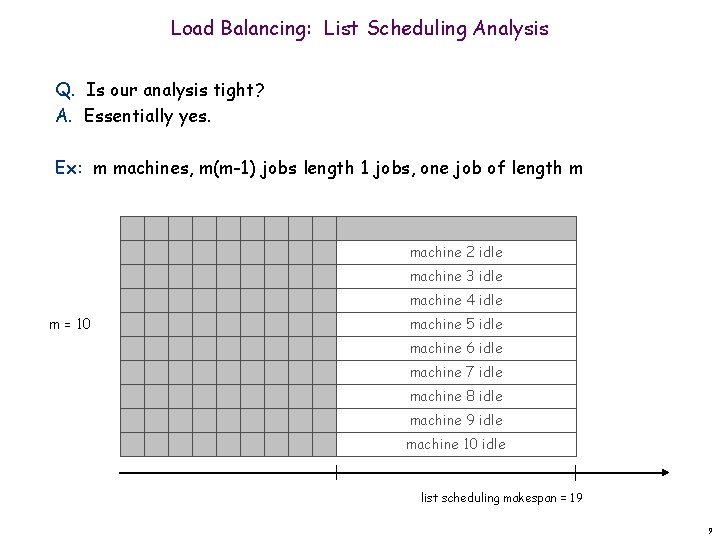Load Balancing: List Scheduling Analysis Q. Is our analysis tight? A. Essentially yes. Ex: m machines, m(m-1) jobs length 1 jobs, one job of length m machine 2 idle machine 3 idle machine 4 idle m = 10 machine 5 idle machine 6 idle machine 7 idle machine 8 idle machine 9 idle machine 10 idle list scheduling makespan = 19 9Load Balancing: List Scheduling Analysis Q. Is our analysis tight? A. Essentially yes. Ex: m machines, m(m-1) jobs length 1 jobs, one job of length m m = 10 optimal makespan = 10 10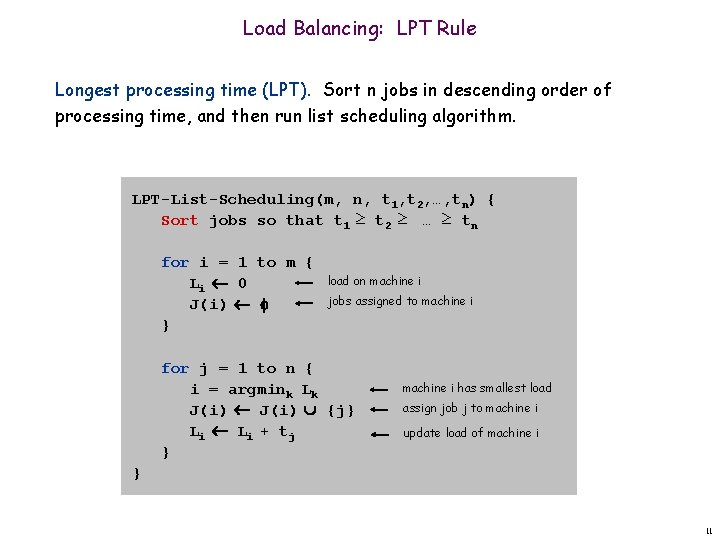Load Balancing: LPT Rule Longest processing time (LPT). Sort n jobs in descending order of processing time, and then run list scheduling algorithm. LPT-List-Scheduling(m, n, t 1, t 2, …, tn) { Sort jobs so that t 1 ≥ t 2 ≥ … ≥ tn for i = 1 to m { Li 0 J(i) } load on machine i jobs assigned to machine i for j = 1 to n { i = argmink Lk J(i) {j} L i + tj } machine i has smallest load assign job j to machine i update load of machine i } 11Load Balancing: LPT Rule Observation. If at most m jobs, then list-scheduling is optimal. Pf. Each job put on its own machine. ▪ Lemma 3. If there are more than m jobs, L* 2 tm+1. Pf. Consider first m+1 jobs t 1, …, tm+1. Since the ti's are in descending order, each takes at least tm+1 time. There are m+1 jobs and m machines, so by pigeonhole principle, at least one machine gets two jobs. ▪ n n n Theorem. LPT rule is a 3/2 approximation algorithm. Pf. Same basic approach as for list scheduling. ▪ Lemma 3 ( by observation, can assume number of jobs > m ) 12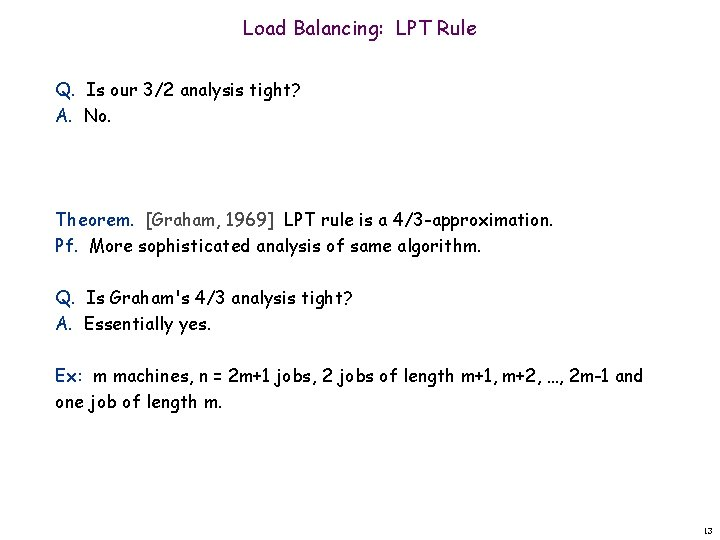Load Balancing: LPT Rule Q. Is our 3/2 analysis tight? A. No. Theorem. [Graham, 1969] LPT rule is a 4/3 -approximation. Pf. More sophisticated analysis of same algorithm. Q. Is Graham's 4/3 analysis tight? A. Essentially yes. Ex: m machines, n = 2 m+1 jobs, 2 jobs of length m+1, m+2, …, 2 m-1 and one job of length m. 1311. 2 Center Selection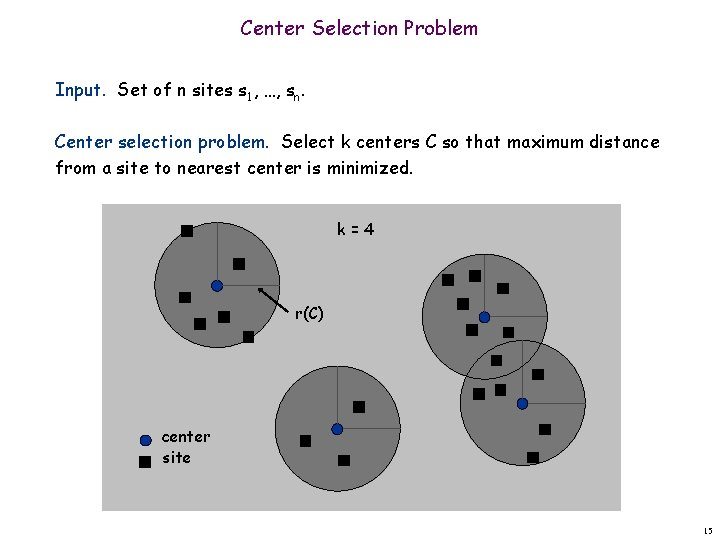Center Selection Problem Input. Set of n sites s 1, …, sn. Center selection problem. Select k centers C so that maximum distance from a site to nearest center is minimized. k=4 r(C) center site 15Center Selection Problem Input. Set of n sites s 1, …, sn. Center selection problem. Select k centers C so that maximum distance from a site to nearest center is minimized. Notation. dist(x, y) = distance between x and y. dist(si, C) = min c C dist(si, c) = distance from si to closest center. r(C) = maxi dist(si, C) = smallest covering radius. n n n Goal. Find set of centers C that minimizes r(C), subject to |C| = k. Distance function properties. dist(x, x) = 0 dist(x, y) = dist(y, x) dist(x, y) dist(x, z) + dist(z, y) n n n (identity) (symmetry) (triangle inequality) 16Center Selection Example Ex: each site is a point in the plane, a center can be any point in the plane, dist(x, y) = Euclidean distance. Remark: search can be infinite! r(C) center site 17Greedy Algorithm: A False Start Greedy algorithm. Put the first center at the best possible location for a single center, and then keep adding centers so as to reduce the covering radius each time by as much as possible. Remark: arbitrarily bad! greedy center 1 k = 2 centers center site 18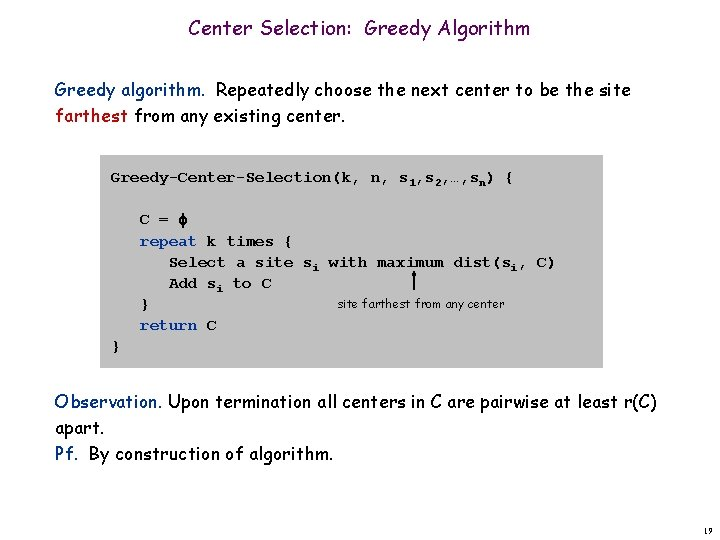Center Selection: Greedy Algorithm Greedy algorithm. Repeatedly choose the next center to be the site farthest from any existing center. Greedy-Center-Selection(k, n, s 1, s 2, …, sn) { C = repeat k times { Select a site si with maximum dist(si, C) Add si to C site farthest from any center } return C } Observation. Upon termination all centers in C are pairwise at least r(C) apart. Pf. By construction of algorithm. 19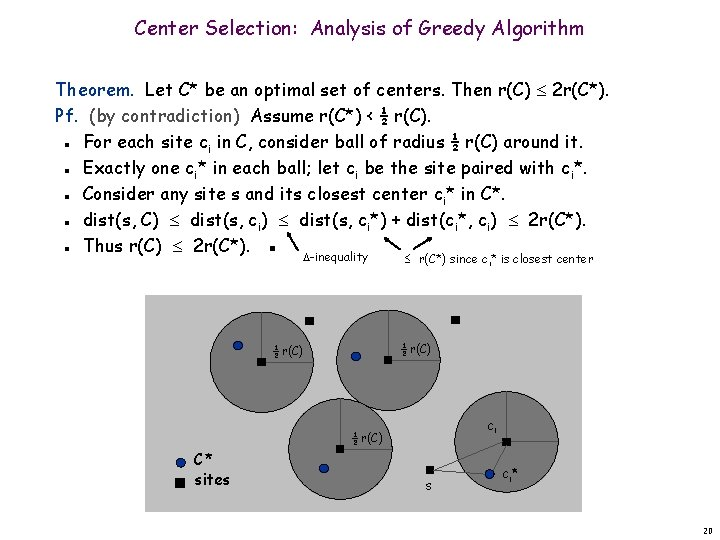Center Selection: Analysis of Greedy Algorithm Theorem. Let C* be an optimal set of centers. Then r(C) 2 r(C*). Pf. (by contradiction) Assume r(C*) < ½ r(C). For each site ci in C, consider ball of radius ½ r(C) around it. Exactly one ci* in each ball; let ci be the site paired with ci*. Consider any site s and its closest center ci* in C*. dist(s, C) dist(s, ci*) + dist(ci*, ci) 2 r(C*). Thus r(C) 2 r(C*). ▪ n n n -inequality r(C*) since ci* is closest center ½ r(C) ci ½ r(C) C* sites s ci * 20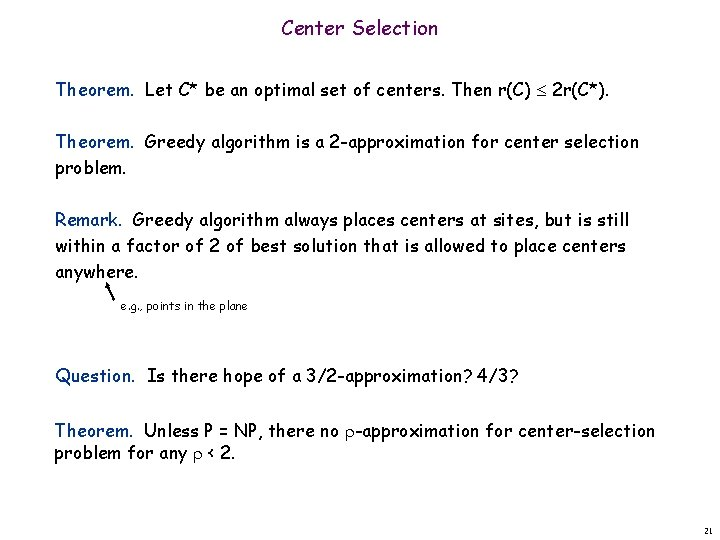Center Selection Theorem. Let C* be an optimal set of centers. Then r(C) 2 r(C*). Theorem. Greedy algorithm is a 2 -approximation for center selection problem. Remark. Greedy algorithm always places centers at sites, but is still within a factor of 2 of best solution that is allowed to place centers anywhere. e. g. , points in the plane Question. Is there hope of a 3/2 -approximation? 4/3? Theorem. Unless P = NP, there no -approximation for center-selection problem for any < 2. 21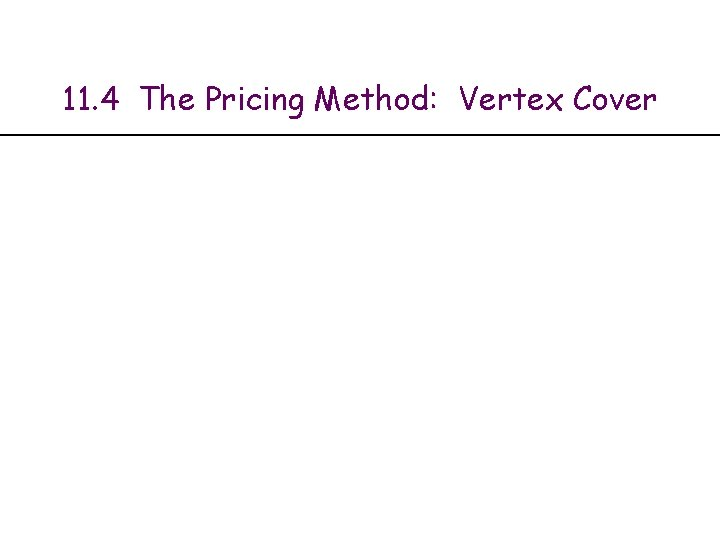11. 4 The Pricing Method: Vertex CoverWeighted Vertex Cover Weighted vertex cover. Given a graph G with vertex weights, find a vertex cover of minimum weight. 2 4 2 9 weight = 2 + 4 weight = 9 23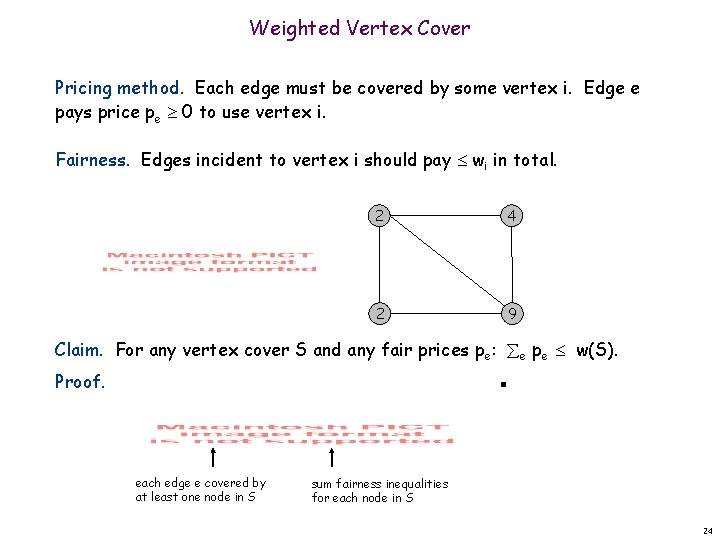Weighted Vertex Cover Pricing method. Each edge must be covered by some vertex i. Edge e pays price pe 0 to use vertex i. Fairness. Edges incident to vertex i should pay wi in total. 2 4 2 9 Claim. For any vertex cover S and any fair prices pe: e pe w(S). Proof. ▪ each edge e covered by at least one node in S sum fairness inequalities for each node in S 24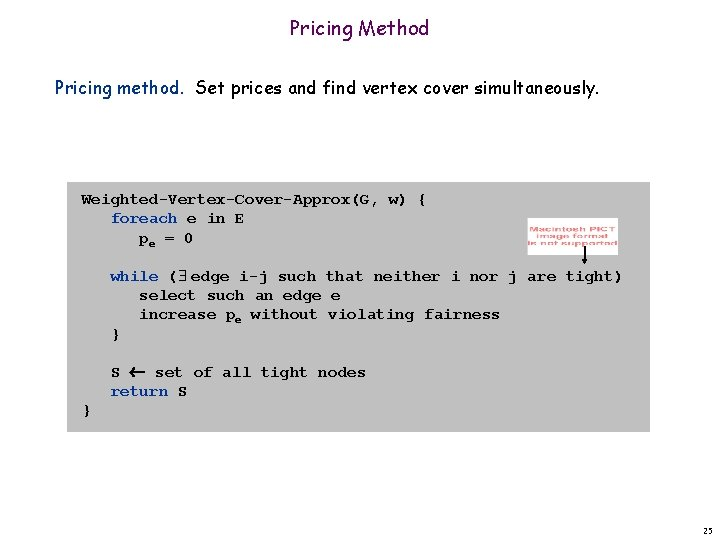Pricing Method Pricing method. Set prices and find vertex cover simultaneously. Weighted-Vertex-Cover-Approx(G, w) { foreach e in E pe = 0 while ( edge i-j such that neither i nor j are tight) select such an edge e increase pe without violating fairness } S set of all tight nodes return S } 25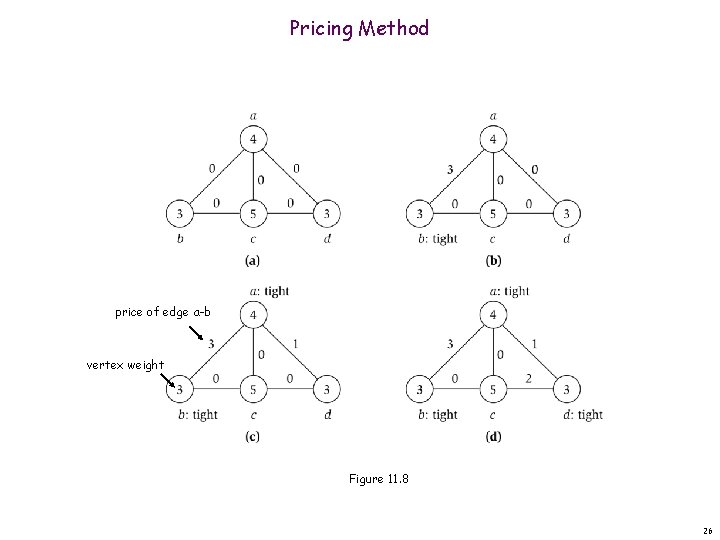Pricing Method price of edge a-b vertex weight Figure 11. 8 26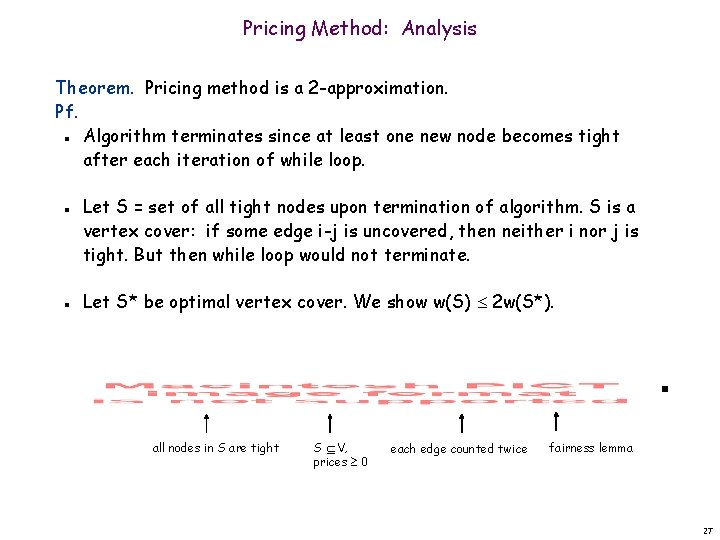Pricing Method: Analysis Theorem. Pricing method is a 2 -approximation. Pf. Algorithm terminates since at least one new node becomes tight after each iteration of while loop. n n n Let S = set of all tight nodes upon termination of algorithm. S is a vertex cover: if some edge i-j is uncovered, then neither i nor j is tight. But then while loop would not terminate. Let S* be optimal vertex cover. We show w(S) 2 w(S*). all nodes in S are tight S V, prices 0 each edge counted twice fairness lemma 2711. 6 LP Rounding: Vertex CoverWeighted Vertex Cover Weighted vertex cover. Given an undirected graph G = (V, E) with vertex weights wi 0, find a minimum weight subset of nodes S such that every edge is incident to at least one vertex in S. 10 A F 6 9 16 B G 7 10 6 C 3 H 9 23 D I 33 7 E J 10 32 total weight = 55 29Weighted Vertex Cover: IP Formulation Weighted vertex cover. Given an undirected graph G = (V, E) with vertex weights wi 0, find a minimum weight subset of nodes S such that every edge is incident to at least one vertex in S. Integer programming formulation. Model inclusion of each vertex i using a 0/1 variable xi. n Vertex covers in 1 -1 correspondence with 0/1 assignments: S = {i V : xi = 1} n n Objective function: maximize i wi xi. Must take either i or j: xi + xj 1. 30Weighted Vertex Cover: IP Formulation Weighted vertex cover. Integer programming formulation. Observation. If x* is optimal solution to (ILP), then S = {i V : x*i = 1} is a min weight vertex cover. 31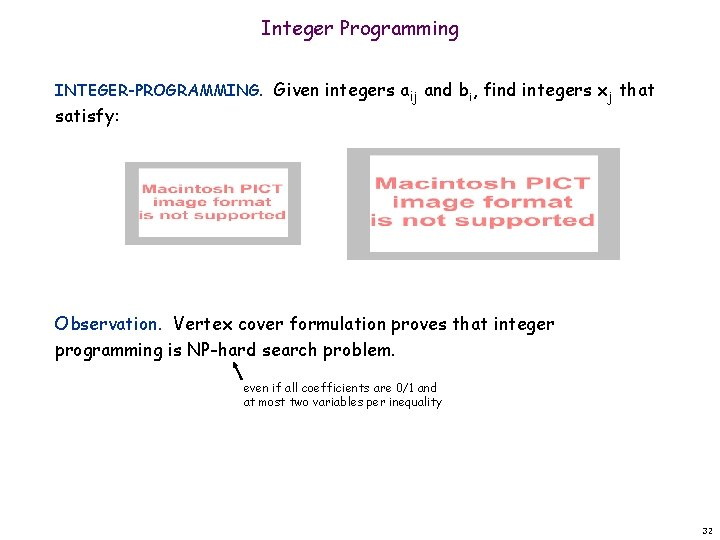Integer Programming INTEGER-PROGRAMMING. Given integers aij and bi, find integers xj that satisfy: Observation. Vertex cover formulation proves that integer programming is NP-hard search problem. even if all coefficients are 0/1 and at most two variables per inequality 32Linear Programming Linear programming. Max/min linear objective function subject to linear inequalities. Input: integers cj, bi, aij. Output: real numbers xj. n n Linear. No x 2, xy, arccos(x), x(1 -x), etc. Simplex algorithm. [Dantzig 1947] Can solve LP in practice. Ellipsoid algorithm. [Khachian 1979] Can solve LP in poly-time. 33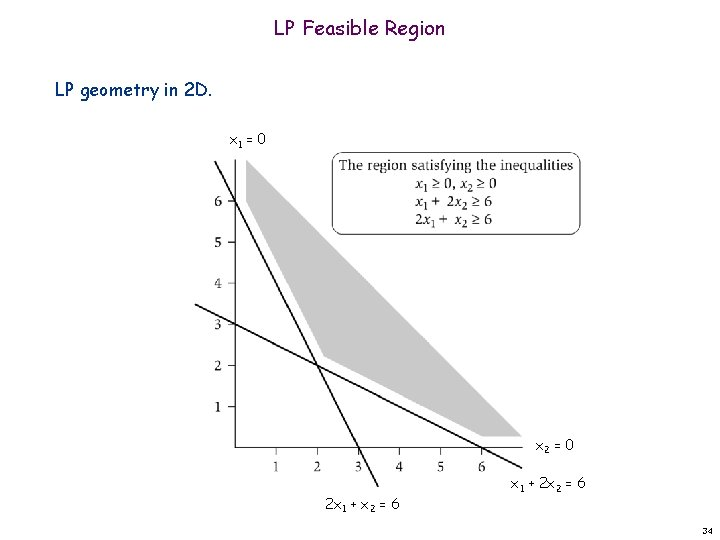LP Feasible Region LP geometry in 2 D. x 1 = 0 x 2 = 0 2 x 1 + x 2 = 6 x 1 + 2 x 2 = 6 34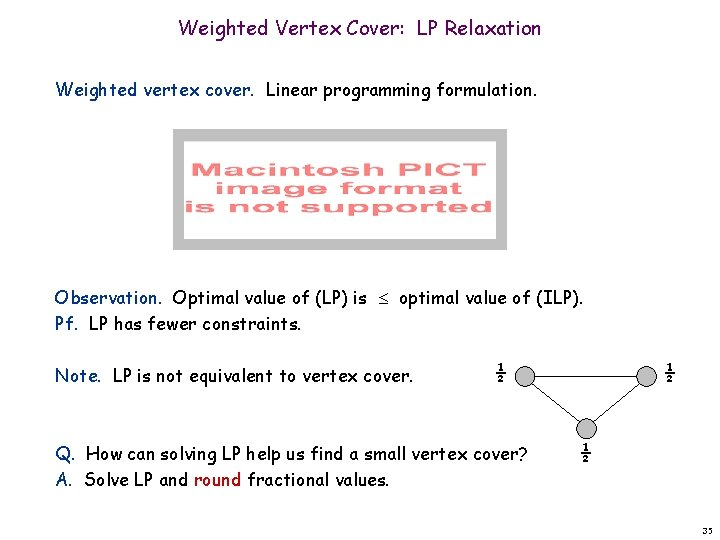Weighted Vertex Cover: LP Relaxation Weighted vertex cover. Linear programming formulation. Observation. Optimal value of (LP) is optimal value of (ILP). Pf. LP has fewer constraints. Note. LP is not equivalent to vertex cover. ½ Q. How can solving LP help us find a small vertex cover? A. Solve LP and round fractional values. ½ ½ 35Weighted Vertex Cover Theorem. If x* is optimal solution to (LP), then S = {i V : x*i ½} is a vertex cover whose weight is at most twice the min possible weight. Pf. [S is a vertex cover] Consider an edge (i, j) E. Since x*i + x*j 1, either x*i ½ or x*j ½ (i, j) covered. n n Pf. [S has desired cost] Let S* be optimal vertex cover. Then n LP is a relaxation x*i ½ 36Weighted Vertex Cover Theorem. 2 -approximation algorithm for weighted vertex cover. Theorem. [Dinur-Safra 2001] If P NP, then no -approximation for < 1. 3607, even with unit weights. 10 5 - 21 Open research problem. Close the gap. 37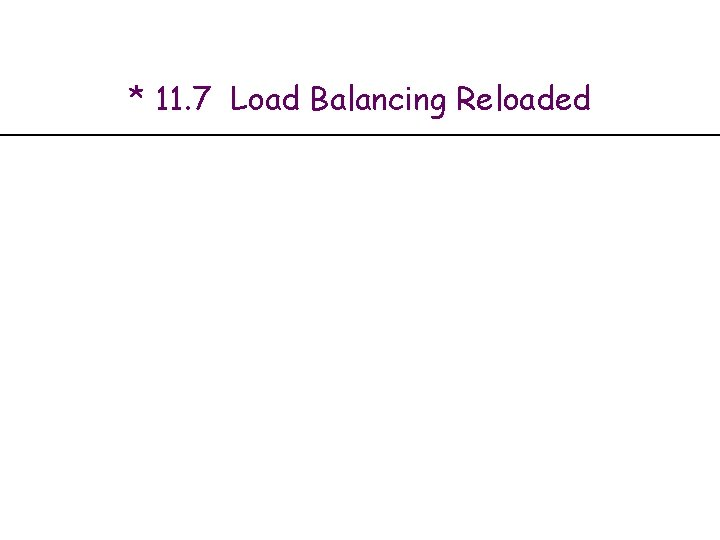Generalized Load Balancing Input. Set of m machines M; set of n jobs J. Job j must run contiguously on an authorized machine in Mj M. Job j has processing time tj. Each machine can process at most one job at a time. n n n Def. Let J(i) be the subset of jobs assigned to machine i. The load of machine i is Li = j J(i) tj. Def. The makespan is the maximum load on any machine = max i Li. Generalized load balancing. Assign each job to an authorized machine to minimize makespan. 39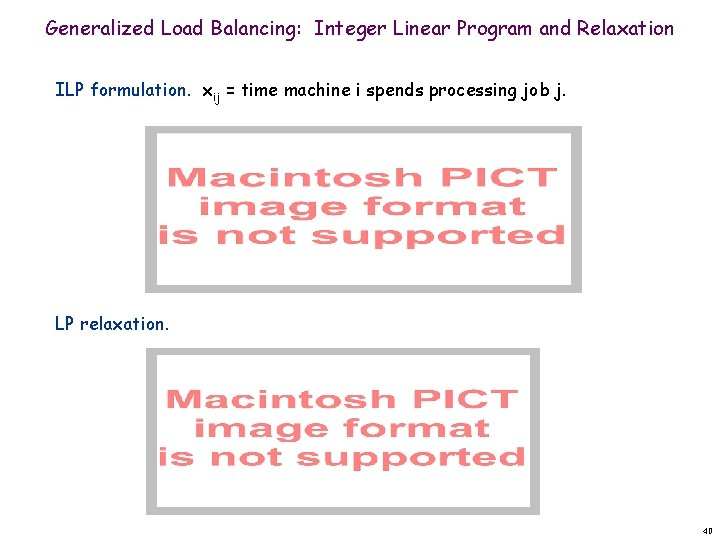Generalized Load Balancing: Integer Linear Program and Relaxation ILP formulation. xij = time machine i spends processing job j. LP relaxation. 40Generalized Load Balancing: Lower Bounds Lemma 1. Let L be the optimal value to the LP. Then, the optimal makespan L* L. Pf. LP has fewer constraints than IP formulation. Lemma 2. The optimal makespan L* maxj tj. Pf. Some machine must process the most time-consuming job. ▪ 41Generalized Load Balancing: Structure of LP Solution Lemma 3. Let x be solution to LP. Let G(x) be the graph with an edge from machine i to job j if xij > 0. Then G(x) is acyclic. can transform x into another LP solution where G(x) is acyclic if LP solver doesn't return such an x Pf. (deferred) xij > 0 G(x) acyclic job G(x) cyclic machine 42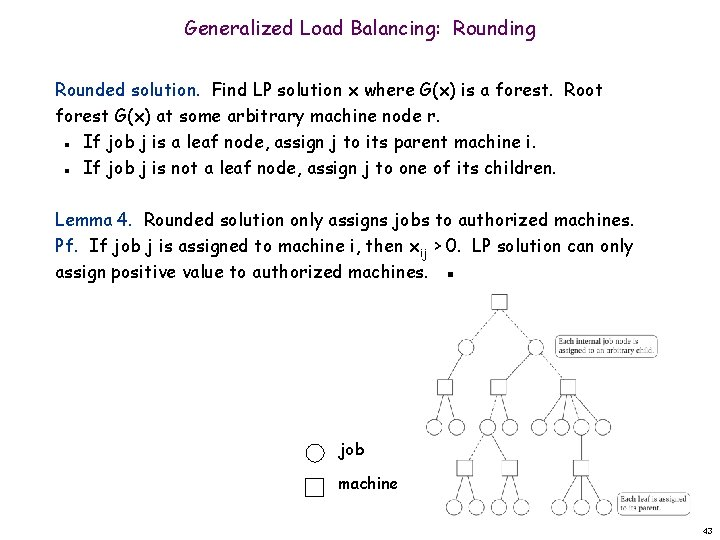Generalized Load Balancing: Rounding Rounded solution. Find LP solution x where G(x) is a forest. Root forest G(x) at some arbitrary machine node r. If job j is a leaf node, assign j to its parent machine i. If job j is not a leaf node, assign j to one of its children. n n Lemma 4. Rounded solution only assigns jobs to authorized machines. Pf. If job j is assigned to machine i, then xij > 0. LP solution can only assign positive value to authorized machines. ▪ job machine 43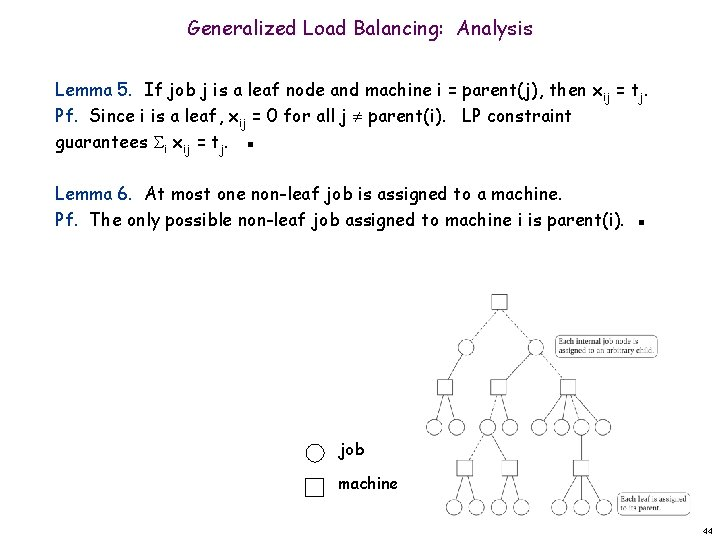Generalized Load Balancing: Analysis Lemma 5. If job j is a leaf node and machine i = parent(j), then xij = tj. Pf. Since i is a leaf, xij = 0 for all j parent(i). LP constraint guarantees i xij = tj. ▪ Lemma 6. At most one non-leaf job is assigned to a machine. Pf. The only possible non-leaf job assigned to machine i is parent(i). ▪ job machine 44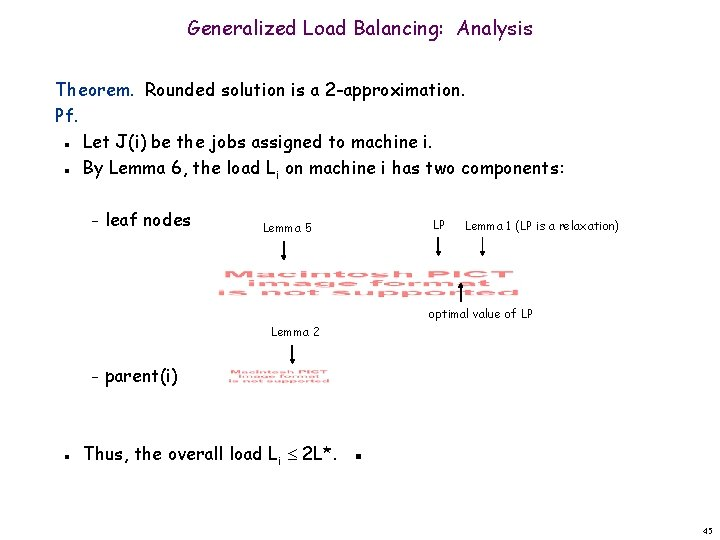Generalized Load Balancing: Analysis Theorem. Rounded solution is a 2 -approximation. Pf. Let J(i) be the jobs assigned to machine i. By Lemma 6, the load Li on machine i has two components: n n – leaf nodes Lemma 5 LP Lemma 1 (LP is a relaxation) optimal value of LP Lemma 2 – n parent(i) Thus, the overall load Li 2 L*. ▪ 45Generalized Load Balancing: Flow Formulation Flow formulation of LP. Observation. Solution to feasible flow problem with value L are in oneto-one correspondence with LP solutions of value L. 46Generalized Load Balancing: Structure of Solution Lemma 3. Let (x, L) be solution to LP. Let G(x) be the graph with an edge from machine i to job j if xij > 0. We can find another solution (x', L) such that G(x') is acyclic. Pf. Let C be a cycle in G(x). flow conservation maintained Augment flow along the cycle C. At least one edge from C is removed (and none are added). Repeat until G(x') is acyclic. n n n 3 3 6 4 2 3 1 5 1 4 6 5 4 3 G(x) augment along C 4 G(x') 47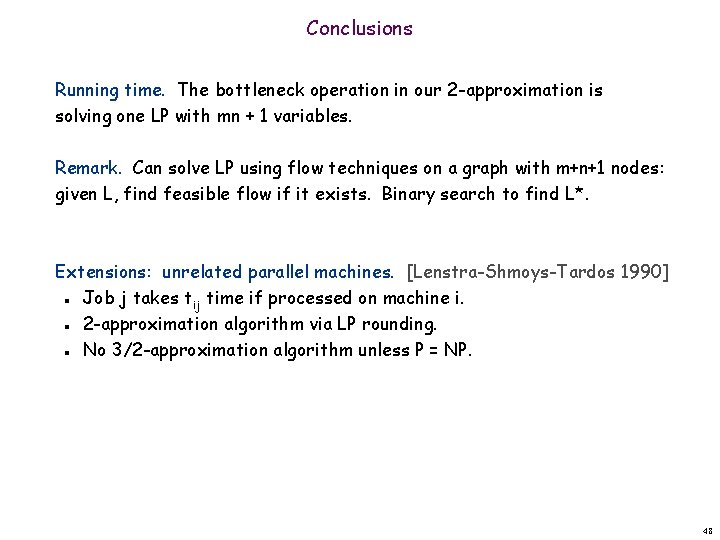Conclusions Running time. The bottleneck operation in our 2 -approximation is solving one LP with mn + 1 variables. Remark. Can solve LP using flow techniques on a graph with m+n+1 nodes: given L, find feasible flow if it exists. Binary search to find L*. Extensions: unrelated parallel machines. [Lenstra-Shmoys-Tardos 1990] Job j takes tij time if processed on machine i. 2 -approximation algorithm via LP rounding. No 3/2 -approximation algorithm unless P = NP. n n n 48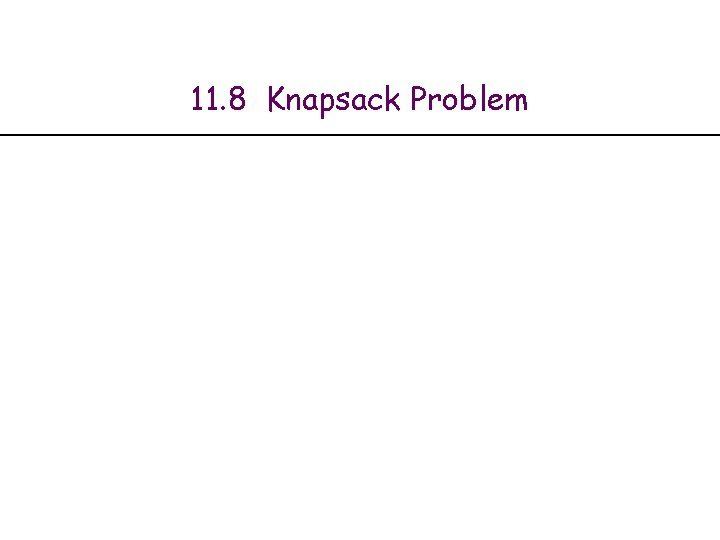11. 8 Knapsack Problem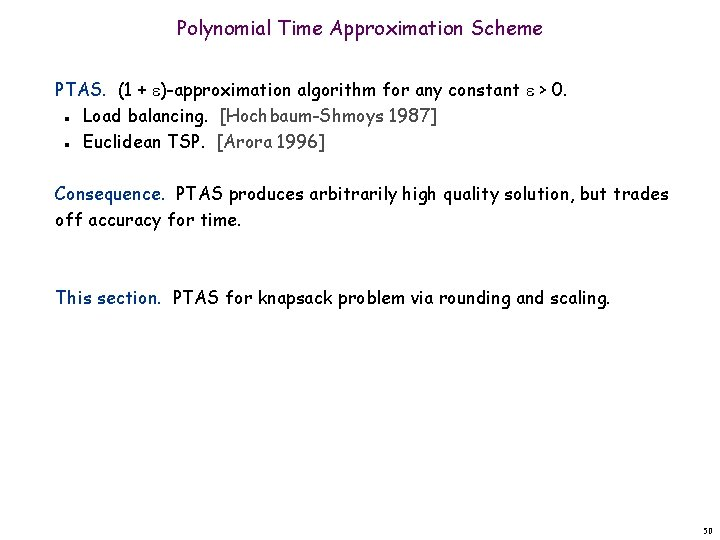Polynomial Time Approximation Scheme PTAS. (1 + )-approximation algorithm for any constant > 0. Load balancing. [Hochbaum-Shmoys 1987] Euclidean TSP. [Arora 1996] n n Consequence. PTAS produces arbitrarily high quality solution, but trades off accuracy for time. This section. PTAS for knapsack problem via rounding and scaling. 50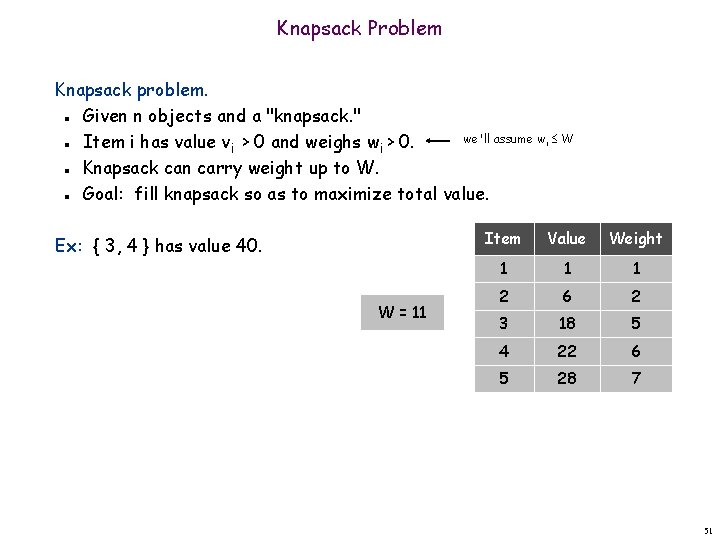Knapsack Problem Knapsack problem. Given n objects and a "knapsack. " we'll assume wi W Item i has value vi > 0 and weighs wi > 0. Knapsack can carry weight up to W. Goal: fill knapsack so as to maximize total value. n n Ex: { 3, 4 } has value 40. W = 11 Item Value Weight 1 1 1 2 6 2 3 18 5 4 22 6 5 28 7 51Knapsack is NP-Complete KNAPSACK: Given a finite set X, nonnegative weights wi, nonnegative values vi, a weight limit W, and a target value V, is there a subset S X such that: SUBSET-SUM: Given a finite set X, nonnegative values ui, and an integer U, is there a subset S X whose elements sum to exactly U? Claim. SUBSET-SUM P KNAPSACK. Pf. Given instance (u 1, …, un, U) of SUBSET-SUM, create KNAPSACK instance: 52Knapsack Problem: Dynamic Programming 1 Def. OPT(i, w) = max value subset of items 1, . . . , i with weight limit w. Case 1: OPT does not select item i. – OPT selects best of 1, …, i– 1 using up to weight limit w Case 2: OPT selects item i. – new weight limit = w – wi – OPT selects best of 1, …, i– 1 using up to weight limit w – wi n n Running time. O(n W). W = weight limit. Not polynomial in input size! n n 53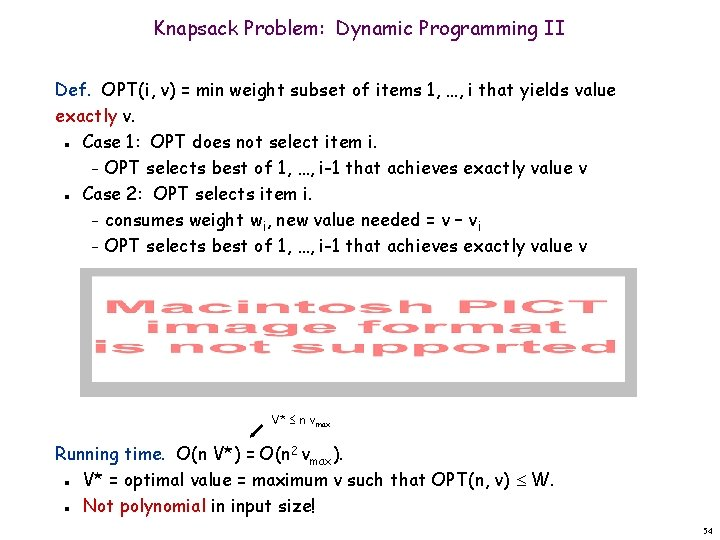Knapsack Problem: Dynamic Programming II Def. OPT(i, v) = min weight subset of items 1, …, i that yields value exactly v. Case 1: OPT does not select item i. – OPT selects best of 1, …, i-1 that achieves exactly value v Case 2: OPT selects item i. – consumes weight wi, new value needed = v – vi – OPT selects best of 1, …, i-1 that achieves exactly value v n n V* n vmax Running time. O(n V*) = O(n 2 vmax). V* = optimal value = maximum v such that OPT(n, v) W. Not polynomial in input size! n n 54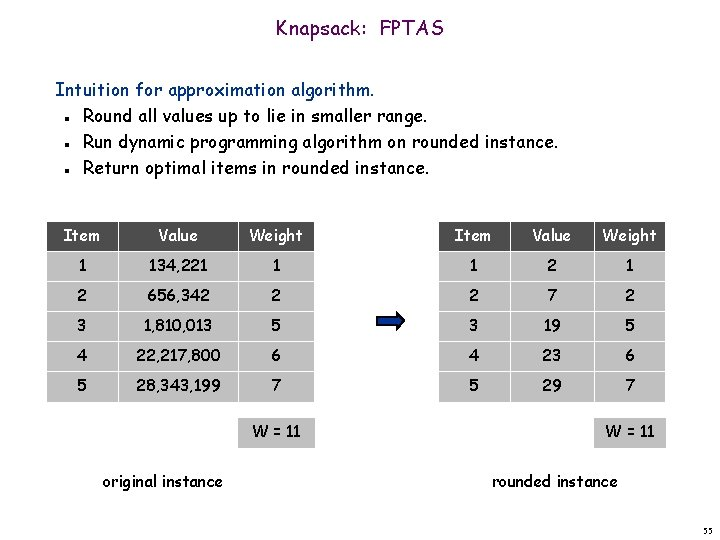Knapsack: FPTAS Intuition for approximation algorithm. Round all values up to lie in smaller range. Run dynamic programming algorithm on rounded instance. Return optimal items in rounded instance. n n n Item Value Weight 1 134, 221 1 1 2 656, 342 2 2 7 2 3 1, 810, 013 5 3 19 5 4 22, 217, 800 6 4 23 6 5 28, 343, 199 7 5 29 7 W = 11 original instance W = 11 rounded instance 55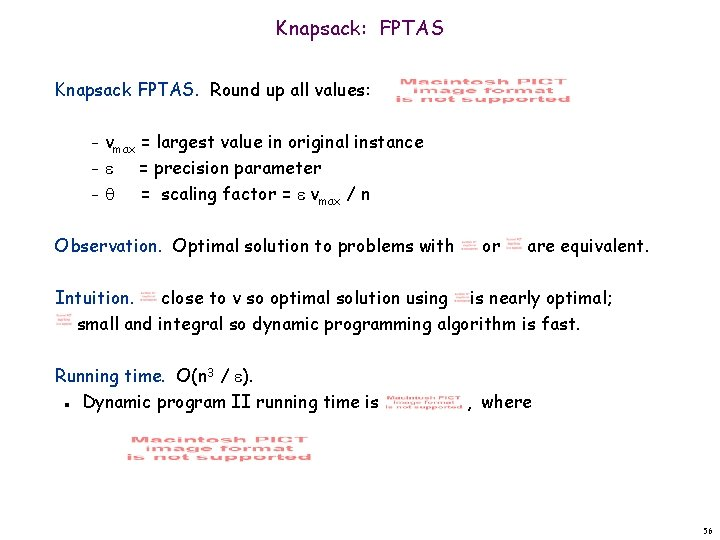Knapsack: FPTAS Knapsack FPTAS. Round up all values: vmax = largest value in original instance – = precision parameter – = scaling factor = vmax / n – Observation. Optimal solution to problems with or are equivalent. Intuition. close to v so optimal solution using is nearly optimal; small and integral so dynamic programming algorithm is fast. Running time. O(n 3 / ). Dynamic program II running time is n , where 56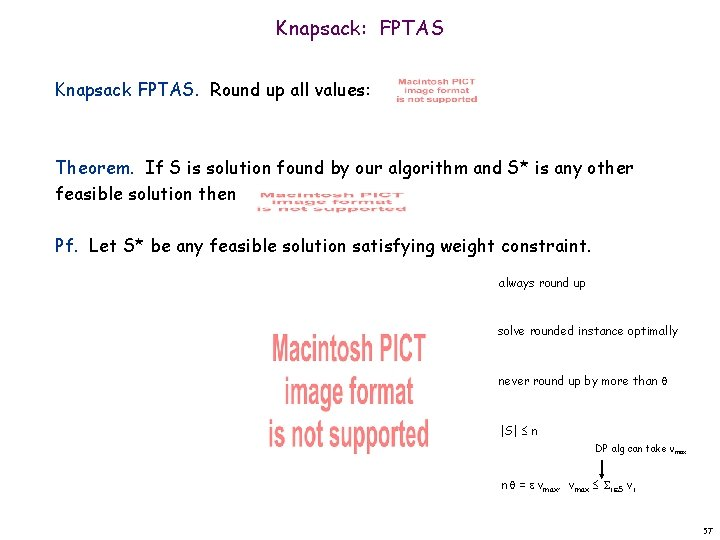Knapsack: FPTAS Knapsack FPTAS. Round up all values: Theorem. If S is solution found by our algorithm and S* is any other feasible solution then Pf. Let S* be any feasible solution satisfying weight constraint. always round up solve rounded instance optimally never round up by more than |S| n DP alg can take vmax n = vmax, vmax i S vi 57Extra Slides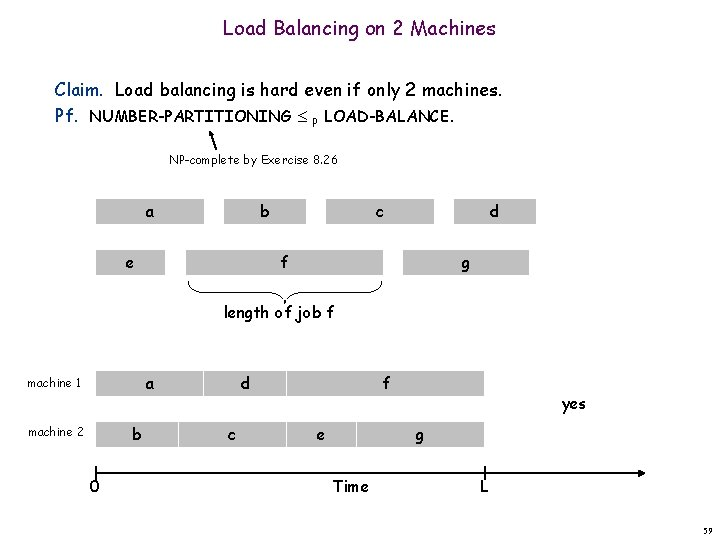Load Balancing on 2 Machines Claim. Load balancing is hard even if only 2 machines. Pf. NUMBER-PARTITIONING P LOAD-BALANCE. NP-complete by Exercise 8. 26 a e b c d f g length of job f a machine 1 b machine 2 0 d Machine 1 f c Machine e 2 yes g Time L 59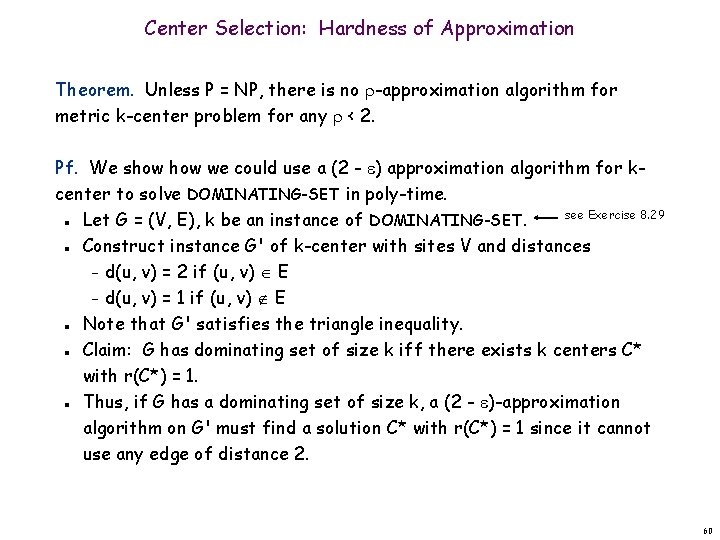Center Selection: Hardness of Approximation Theorem. Unless P = NP, there is no -approximation algorithm for metric k-center problem for any < 2. Pf. We show we could use a (2 - ) approximation algorithm for kcenter to solve DOMINATING-SET in poly-time. see Exercise 8. 29 Let G = (V, E), k be an instance of DOMINATING-SET. Construct instance G' of k-center with sites V and distances – d(u, v) = 2 if (u, v) E – d(u, v) = 1 if (u, v) E Note that G' satisfies the triangle inequality. Claim: G has dominating set of size k iff there exists k centers C* with r(C*) = 1. Thus, if G has a dominating set of size k, a (2 - )-approximation algorithm on G' must find a solution C* with r(C*) = 1 since it cannot use any edge of distance 2. n n n 60